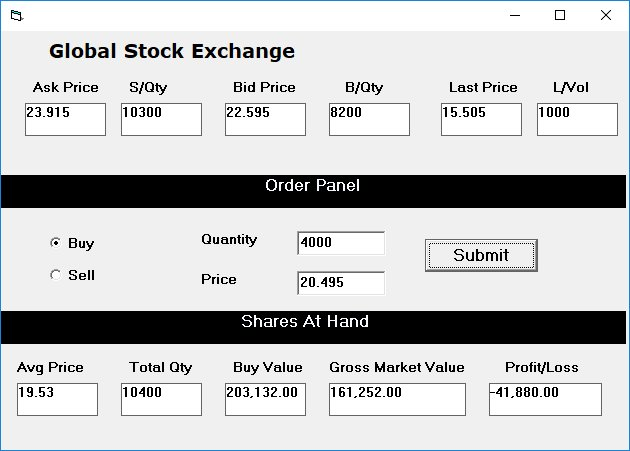You can create a stock trading simulation program easily in Visual Basic. We will create such program using Visual Basic 6. Of course, you can also create the program using other versions of Visual Basic.

For stock trading, we need to consider the following data:

• Asking Price- Price offered by the sellers
• Bidding Price- Price offered by the buyers
• Selling Quantity-Total number of shares available for sale on the stock market
• Buying Quantity- Total number of shares the buyers bid on the stock market
• Last Done Price- The price for the last transaction
• Order Price – Price bid by the trader to buy or to sell
• Order Quantity- Number of Shares ordered by the trader
• Average Price- Average share price
• Buy Value – Average value of shares paid by the  trader
• Gross Market Value- The current market of shares owned by the trader
• The total number of shares in the hand of the trader.
• Profit or Loss

We shall use the following variables to represent the aforementioned data:

BD- Bidding Price
SQ- Selling Quantity
LP- Last Done Price
OP- Order Price
OQ- Order Quantity
AVP- Average Price
MV- Gross Market Value
TQ- Total Number of Shares in Hand

In this program, we need to insert a timer to generate random values for the Asking Price, the Bidding Price, the Selling Quantity, the Buying Quantity and the Last Done Price. You can set the timer to any interval you think is suitable, here we set it to 15 seconds so that the values changes every 15 seconds to simulate the real stock market. The code is as follows:

ranNum1 = Rnd() * 2 + 3
ranNum2 = Rnd() * 2 + 3
ranNum3 = Rnd() * 2 + 3
AP = Round(ranNum1, 3) * 5
SQ = Int(Round(Rnd(), 2) * 10000) + 1000
BD = Round(ranNum2, 3) * 5
BQ = Int(Round(Rnd(), 2) * 10000) + 1000
LP = Round(ranNum3, 3) * 5 ‘Last Done Price
LVOL = Int(Round(Rnd(), 2) * 10000) + 1000 ‘Last Done Volume
PL- Profit or Loss

Besides that, we need to write code to calculate the Average Price, the Buy Value, the Gross Market Value and the total number of shares in hand, as follows:

AVP = (AVP * TQ + OP * OQ) / (TQ + OQ)
TQ = TQ + OQ
MV = LP * TQ
BV = TQ * AVP### The Code

```Dim ranNum1, ranNum2, randNum3, ranNum4 As Double
Dim AP As Single
Dim SQ As Integer
Dim BD As Single
Dim BQ As Integer
Dim OQ As Long
Dim OP As Single
Dim LP As Single
Dim LVOL As Integer
Dim AVP As Single
Dim TQ As Long
Dim BV As Single
Dim MV As Single
Dim PL As Single

Private Sub Cmd_Submit_Click()
OQ = Val(Txt_Quantity.Text)
OP = Val(Txt_Price.Text)
LP = Lbl_LP.Caption
If Opt_Buy.Value = True And BV >= 1000 Then
AVP = (AVP * TQ + OP * OQ) / (TQ + OQ)
TQ = TQ + OQ
Lbl_TQ.Caption = TQ
MV = LP * TQ
BV = TQ * AVP
PL = MV - BV
Lbl_LP.Caption = Format(LP, "#,##.00")
Lbl_MV.Caption = Format(MV, "#,##.00")
Lbl_BV.Caption = Format(BV, "#,##.00")
Lbl_PL.Caption = Format(PL, "#,##.00")
Lbl_PL.Caption = Format(PL, "#,##.00")
Lbl_AVP.Caption = Format(AVP, "#,##.00")
ElseIf Opt_Buy.Value = True And BV < 1000 Then MsgBox ("You don't have enough fund to buy, reduce order") End If If Opt_Sell.Value = True And TQ >= OQ Then
AVP = (AVP * TQ + OP * OQ) / (TQ + OQ)
TQ = TQ - OQ
Lbl_TQ.Caption = TQ
MV = LP * TQ
BV = TQ * AVP
PL = MV - BV
Lbl_MV.Caption = Format(MV, "#,##.00")
Lbl_BV.Caption = Format(BV, "#,##.00")
Lbl_PL.Caption = Format(PL, "#,##.00")
Lbl_AVP.Caption = Format(AVP, "#,##.00")

ElseIf Opt_Sell.Value = True And TQ < OQ Then
MsgBox ("Not enough shares, reduce order")
End If

End Sub

Randomize
ranNum1 = Rnd() * 2 + 3
ranNum2 = Rnd() * 2 + 3
ranNum3 = Rnd() * 2 + 3
ranNum4 = Rnd() * 2 + 3
AVP = Round(ranNum1, 3) * 5 ' Average Price
TQ = Int(Round(Rnd(), 2) * 10000) + 1000 'Total Shares at Hand
BV = AVP * TQ 'Buy Value
AP = Round(ranNum2, 3) * 5 'Asking Price
SQ = Int(Round(Rnd(), 2) * 10000) + 1000
BD = Round(ranNum2, 3) * 5 'Bidding Price
BQ = Int(Round(Rnd(), 2) * 10000) + 1000
LP = Round(ranNum2, 3) * 5 'Last Done Price
MV = LP * TQ
PL = MV - BV
LVOL = Int(Round(Rnd(), 2) * 10000) + 1000 'Last Done Volume
Lbl_AP.Caption = AP
Lbl_SQ.Caption = SQ
Lbl_BD.Caption = BD
Lbl_BQ.Caption = BQ
Lbl_LP.Caption = OP
Lbl_AVP.Caption = AVP
Lbl_TQ.Caption = TQ
Lbl_MV.Caption = Format(MV, "#,##.00")
Lbl_PL.Caption = Format(PL, "#,##.00")
Lbl_LP.Caption = Format(LP, "#,##.00")
Lbl_BV.Caption = Format(BV, "#,##.00")
Lbl_Vol.Caption = LVOL

End Sub

Private Sub Timer1_Timer()
Randomize
ranNum1 = Rnd() * 2 + 3
ranNum2 = Rnd() * 2 + 3
ranNum3 = Rnd() * 2 + 3
AP = Round(ranNum1, 3) * 5
SQ = Int(Round(Rnd(), 2) * 10000) + 1000
BD = Round(ranNum2, 3) * 5
BQ = Int(Round(Rnd(), 2) * 10000) + 1000
LP = Round(ranNum3, 3) * 5 'Last Done Price
MV = LP * TQ ' Market Value
PL = MV - BV
LVOL = Int(Round(Rnd(), 2) * 10000) + 1000 'Last Done Volume
Lbl_AP.Caption = AP
Lbl_SQ.Caption = SQ
Lbl_BD.Caption = BD
Lbl_BQ.Caption = BQ
Lbl_LP.Caption = LP
Lbl_Vol.Caption = LVOL
Lbl_MV.Caption = Format(MV, "#,##.00")
Lbl_PL.Caption = Format(PL, "#,##.00")
End Sub

```

### The Runtime Interface